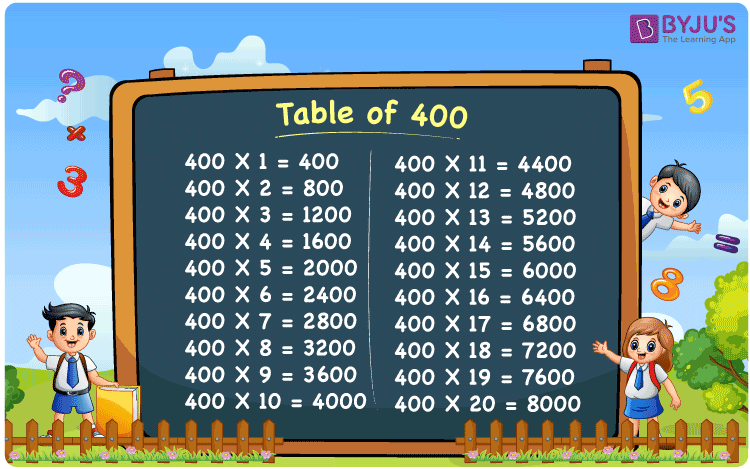Checkout JEE MAINS 2022 Question Paper Analysis : Checkout JEE MAINS 2022 Question Paper Analysis :

# Table of 400

The Multiplication Table for 400 is nothing more than the repetitive addition of the number 400. Repetitive addition is adding the same number multiple times. Memorizing the table for 400 is easy as it is the same as the table for 4 but ending with two zeros. You can find the table of 4 at Table for 4 for comparison.

## Table of 400 Chart## What is the 400 Times Table?

In the table of 400, we are repeatedly adding 400 multiple times. And the table below gives a repetitive addition 10 times for the number 400.

 400 × 1 = 400 400 400 × 2 = 800 400 + 400 = 800 400 × 3 = 1200 400 + 400 + 400 = 1200 400 × 4 = 1600 400 + 400 + 400 + 400 = 1600 400 × 5 = 2000 400 + 400 + 400 + 400 + 400 = 2000 400 × 6 = 2400 400 + 400 + 400 + 400 + 400 + 400 = 2400 400 × 7 = 2800 400 + 400 + 400 + 400 + 400 + 400 + 400 = 2800 400 × 8 = 3200 400 + 400 + 400 + 400 + 400 + 400 + 400 + 400 = 3200 400 × 9 = 3600 400 + 400 + 400 + 400 + 400 + 400 + 400 + 400 + 400 = 3600 400 × 10 = 4000 400 + 400 + 400 + 400 + 400 + 400 + 400 + 400 + 400 + 400 = 4000

## Multiplication Table of 400

Given below is the multiplication table of 400 being done 20 times. The below table helps you with multiplication problems that require the tables of 400.

 400 × 1 = 400 400 × 2 = 800 400 × 3 = 1200 400 × 4 = 1600 400 × 5 = 2000 400 × 6 = 2400 400 × 7 = 2800 400 × 8 = 3200 400 × 9 = 3600 400 × 10 = 4000 400 × 11 = 4400 400 × 12 = 4800 400 × 13 = 5200 400 × 14 = 5600 400 × 15 = 6000 400 × 16 = 6400 400 × 17 = 6800 400 × 18 = 7200 400 × 19 = 7600 400 × 20 = 8000

## Solved Example on Table of 400

Example:

Solve for y: 400 x y = 7200

Solution: y = 18.

400 x y = 7200

y = 7200 / 400 = 18.

## Frequently Asked Questions on Table of 400

### Question 1:What is 100 times 400?

Answer: 100 times 400 is 40000.

### Question 2: Is 4400 a multiple of 400?

Answer: Yes. 4400 is a multiple of 400. 11 times 400 is 4400.

### Question 3: Is 400 a perfect square number?

Answer: Yes. 400 is a perfect square number. 20 times 20 is 400.